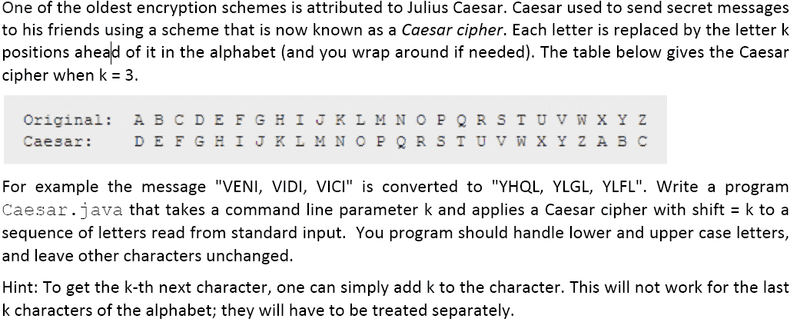# Caesar java program.

• Comp Sci

## Homework Statement## The Attempt at a Solution

Code:
public class Caesar
{
public static void main(String[] args)
{
int k = Integer.parseInt(args);
char c;

System.out.print("Encrypted sentence is: " );

for (int i = 0; i < strMsg.length(); i++)
{
c = strMsg.charAt(i); // get characters
c = (char) (c + k); // shift characters of string k times
System.out.print(c);

}
System.out.println();
}
}

Okay, this works, well almost. Suppose that the shifting value is k = 3, then it will look like the original/caesar in the above image. If I want to decrypt (or encrypt? You get the point.) the message Wxyz, then the output will result in characters different than Zabc. Basically, the 'Hint' part is where I am having trouble making.

And is the code I wrote equivalent to the question/problem asked? I'm not positive I should input a String.

Last edited:

wukunlin
Gold Member
for example, if a correspond to 1, b to 2 etc...
then you can write up a statement saying if c + K >26 then the character becomes ... [what do you do when you are trying to get the "28'th" letter when you actually need the 2nd?]

but this poses a problem on case sensitivity, judging from the difficulty of this exercise you probably don't need to worry about it

Okay, so I was thinking of another way, since we must study both cases(lower- and upper-case). I used the ASCII character to decimal table to do my code..

Code:
public class Caesar
{
public static void main(String[] args)
{
int k = Integer.parseInt(args); // value to shift letters

System.out.print("Enter a message to encrypt: ");

while (!StdIn.isEmpty())
{
for (int i = 0; i < strMsg.length(); i++)
{
char ltr = strMsg.charAt(i);
if (ltr >= 'A' && ltr <= 'Z') // if letters are upper-cased
{
if((int) ltr <= 90 - k)
{
ltr = (char) (ltr + k);
System.out.print(ltr);
}
else
{
ltr = (char) (((int) ltr + k) - 26);
System.out.print(ltr);
}
}
else if (ltr >= 'a' && ltr <= 'z') // if letters are lower-cased
{
if((int) ltr <= 122 - k)
{
ltr = (char) (ltr + k);
System.out.print(ltr);
}
else
{
ltr = (char) (((int) ltr + k) - 26);
System.out.print(ltr);
}
}
else if (!Character.isLetter(ltr)) // if character not letter
{
System.out.print(ltr); // print the character as is
}
}
System.out.print(" ");
}
}
}

In the nested ifs, is there another way for the expression? I don't know if we can use that, but it worked perfectly fine.